UPDATE 2020-11-15: a more complete description of ABC analysis patterns for PowerPivot and Power BI is available in the ABC Classification patterns on DAX Patterns.

The calculation for ABC analysis can be made in PowerPivot using calculated columns. In this way each row can have an attribute with the appropriate ABC class. The ABC calculation has to be made considering a particular grouping and sort order.

For example, we wanted to define an ABC class of Products for internet sales transactions of AdventureWorks. We want to classify our Products in this way:

• Products that make 70% of the sales are in Class A
• Products that make 20% of the sales are in Class B
• Products that make 10% of the sales are in Class C

Example with a single denormalized table

In this first example we use a completely denormalized table. A different approach would be necessary using a normalized product table.

WARNING: Please note that calculating ABC class on a denormalized table could have bad performances because it requires heavy calculation during data load. The normalized approach is far better in terms of performance, because the calculation only depends on the size of the related table (usually the dimension) and not on the size of the table containing the sale transactions.

This is the query we use to get data from AdventureWorks DW.

SELECT
c.FirstName + ‘ ‘ + c.LastName AS Customer,
p.EnglishProductName,
p.ModelName,
s.OrderQuantity,
s.SalesAmount
FROM dbo.FactInternetSales s
INNER JOIN dbo.DimProduct p
ON p.ProductKey = s.ProductKey
INNER JOIN dbo.DimCustomer c
ON s.CustomerKey = c.CustomerKey

There are several steps to obtain the desired ABC class, each of them is a new calculated column in the PowerPivot dataset. Before starting, consider that we will use the ProductName and the SalesAmount columns in our formulas.

• SalesAmountProduct – this is the sales amount for each Product; the same value is duplicated for each row of the same product. The EARLIER function get the product name of the current row and the FILTER returns all the rows for the sales of the same product. This complexity is not required in the normalized version of the ABC calculation.
= SUMX( FILTER( ‘Sales’,
‘Sales'[ProductName] = EARLIER(‘Sales'[ProductName]) ),
‘Sales'[SalesAmount] )
• CumulatedProduct – this value is the cumulated value of a product, considering them ordered from the top-seller down to the worst one. In this case, the EARLIER function is used to get the sales amount for the current product and the FILTER returns all the rows of the products that sold at least the amount value of the current product.
= SUMX( FILTER( ‘Sales’,
‘Sales'[SalesAmountProduct] >=
EARLIER(‘Sales'[SalesAmountProduct] ) ),
‘Sales'[SalesAmount] )
• SortedWeightProduct – This calculation simply transforms the CumulatedProduct calculation into a percentage. This number will be used to filter all the transactions according to the percentage limit corresponding to ABC classes (usually A = 70%, B = 20% and C = 10%).
=’Sales'[CumulatedProduct] / SUM( ‘Sales'[SalesAmount] )
• ABC Class Product – this is the final result of our calculation; depending of the value of SortedWeightProduct an A, B or C is displayed
=IF( ‘Sales'[SortedWeightProduct] < 0.7,
“A”,
IF( ‘Sales'[SortedWeightProduct] < 0.9,
“B”,
“C” ) )

In this way, we obtain an ABC class for each product sale.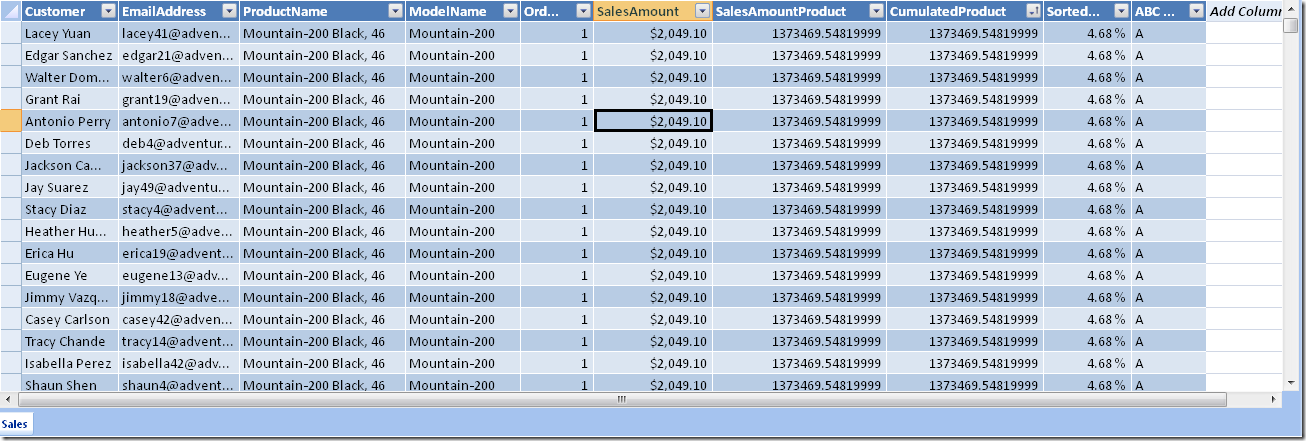At this point, we can browse the data with a PivotTable in Excel. Just to make an example, we can look at the relevance of ABC products for each customer (in this case customers are sorted by total of sales in descending order).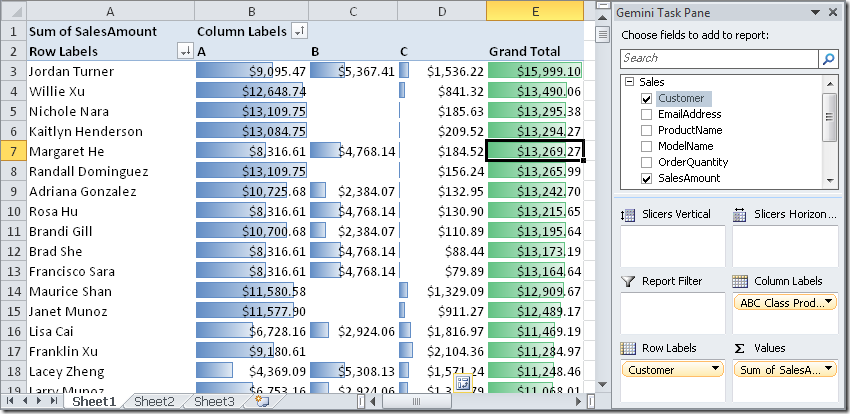Example with normalized tables

In this second example we use three normalized tables, importing from AdventureWorks DW the following tables:

• DimCustomer
• DimProduct
• FactInternetSales

In this case, we defines these calculated columns in the DimProduct table. In this case will use the ProductKey to identify the product and SalesAmount as the measure to use for ABC classification.

• SalesAmountProduct – this is the sales amount for each Product; in this case, each calculation is different for each row, because each row is a single product. We make use of the existing relationship between FactInternetSales and DimProduct (remember that we are defining a calculated column in the DimProduct table).
= SUMX( RELATEDTABLE(‘FactInternetSales’),
‘FactInternetSales'[SalesAmount] )
• CumulatedProduct – this value is the cumulated value of a product, considering them ordered from the top-seller down to the worst one. In this case, the EARLIER function is used to get the sales amount for the current product and the FILTER returns all the rows of the products that sold at least the amount value of the current product.
= SUMX( FILTER( ‘DimProduct’,
‘DimProduct'[SalesAmountProduct] >=
EARLIER( ‘DimProduct'[SalesAmountProduct] ) ),
‘DimProduct'[SalesAmountProduct] )
• SortedWeightProduct – This calculation simply transforms the CumulatedProduct calculation into a percentage. This number will be used to filter all the transactions according to the percentage limit corresponding to ABC classes (usually A = 70%, B = 20% and C = 10%).
= ‘DimProduct'[CumulatedProduct] / SUM( ‘DimProduct'[SalesAmountProduct] )
• ABC Class Product – this is the final result of our calculation; depending of the value of SortedWeightProduct an A, B or C is displayed
= IF( ‘DimProduct'[SortedWeightProduct] < 0.7,
“A”,
IF( ‘DimProduct'[SortedWeightProduct] < 0.9,
“B”,
“C” ) )

As we said, the calculated columns are all defined in the DimProduct table.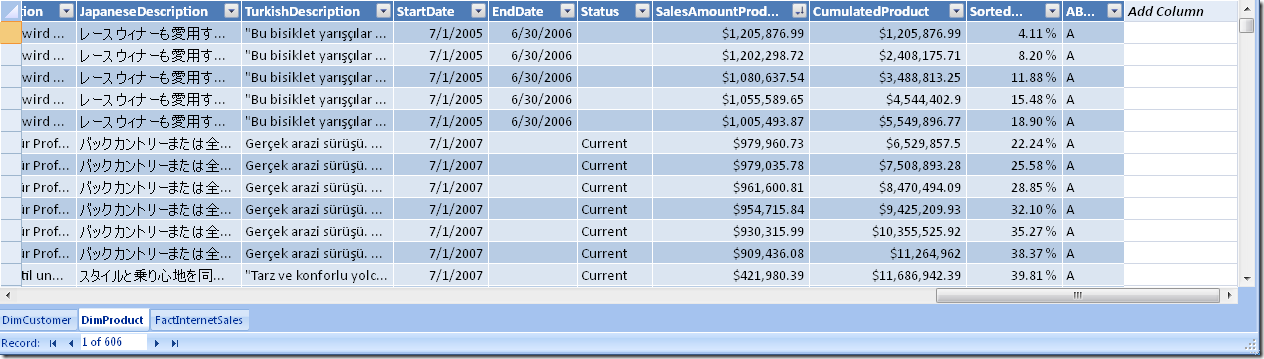Finally, we can do the same ABC analysis as in the case of the denormalized table used as a source of data. In this case we see the distribution of ABC class of products among models. As we might expect, there is a strong relationship, which indicates we might classify Models instead of products, producing a very similar results, but with a few notable exceptions (like the Road-650 model at row 11, which indicates there are smaller sales divided between very similar products). From a business point of view, this could be an important decision to be made (classifying ABC for models instead than for products), especially if products differs only for characteristics that doesn’t affect the production lines.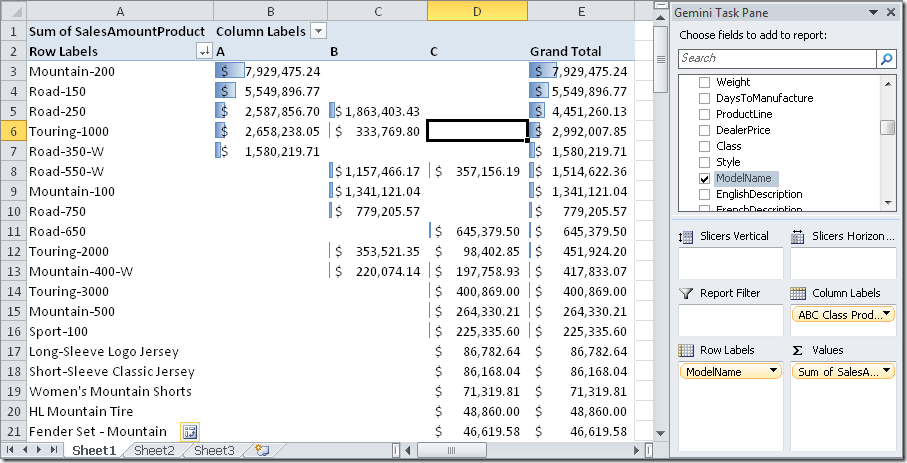Working with denormalized attributes on normalized tables

At this point, it could be interesting to look at how we can write the ABC classification based on the models of the product using the previous example with normalized tables. In this case, we will use a mix of the techniques we have seen in the two scenarios (denormalized and normalized tables).

The set of tables is the same as the previous example and we will continue to add columns to the DimProduct table. In this case, the ModelName column will be our key to identify the granularity of ABC classification.

• SalesAmountModel – this is the sales amount for each product Model; in this case, calculation is the same for all the products of the same model. The EARLIER function get the model name of the current row (a single product) and the FILTER returns all the products of the same model.
=SUMX( FILTER( ‘DimProduct’,
‘DimProduct'[ModelName] =
EARLIER( ‘DimProduct'[ModelName] ) ),
‘DimProduct'[SalesAmountProduct] )
• CumulatedProduct – this value is the cumulated value of a model, considering them ordered from the top-seller down to the worst one. We have to use the CALCULATE function to sum a calculated column filtering only the rows (products) of a certain model. We cannot use the SUMX on the FILTER result, because it wouldn’t contain the SalesAmountProduct column we need to sum.
= SUMX( FILTER( ‘DimProduct’ ,
‘DimProduct'[SalesAmountModel] >=
EARLIER( ‘DimProduct'[SalesAmountModel] )),
‘DimProduct'[SalesAmountProduct] )
• SortedWeightProduct – This calculation simply transforms the CumulatedModel calculation into a percentage. This number will be used to filter all the transactions according to the percentage limit corresponding to ABC classes (usually A = 70%, B = 20% and C = 10%). Please look at the denominatore, which contains an aggregatable column (SalesAmountProduct) instead of the sum of the CumulatedModel column, which is not aggregatable!
= ‘DimProduct'[CumulatedModel] / SUM( ‘DimProduct'[SalesAmountProduct] )
• ABC Class Product – this is the final result of our calculation; depending of the value of SortedWeightProduct an A, B or C is displayed
= IF( ‘DimProduct'[SortedWeightModel] < 0.7,
“A”,
IF( ‘DimProduct'[SortedWeightModel] < 0.9,
“B”,
“C” ) )

This is the new DimProduct with both the ABC Class Product and ABC Class Model (even if it is not visible in this screenshot, there are several cases where these two calculated columns don’t have the same value).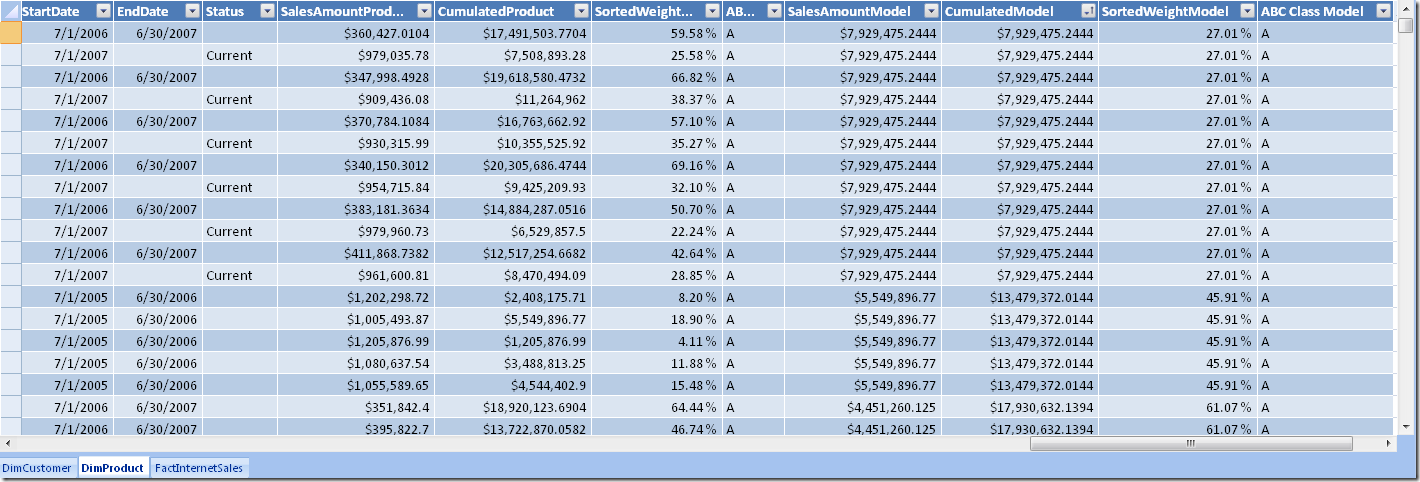Using the ABC Class Model, we can see that each model only belongs to one class.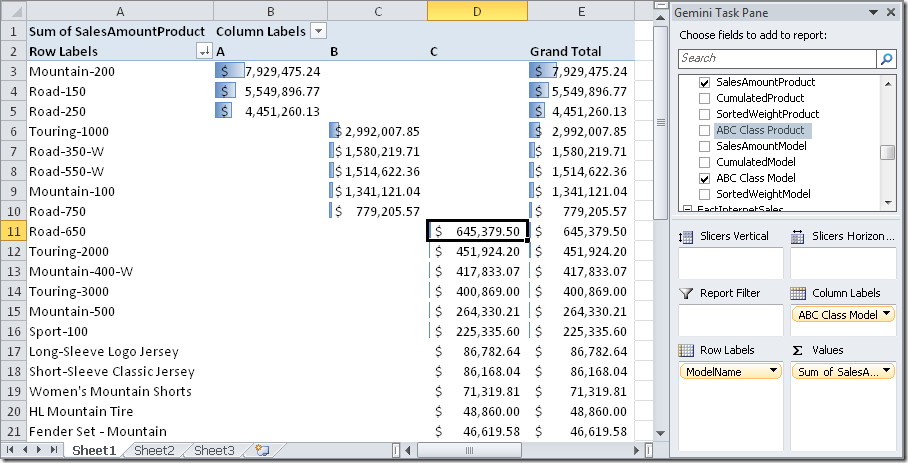Common calculations

Coming back to DAX, we can see that the three cases are very similar and, just to simplify cut & paste of these calculations, we can customize just the first column, leaving standard DAX formula (without the name of the table) in the following ones. Usually, we will need to create just one ABC class in a PowerPivot workbook. For this reason, having these formulas ready to use could be useful. I use the example using SalesAmountProduct as a starting measure (we can simply search & replace this name with our own measure) and ‘Table’ to indicate the table in which we create these calculated columns with the data in PowerPivot.

• SalesAmountProduct – this is the only calculation that differs for each implementation. Look at previous examples to check what DAX formula to use.
• CumulatedProduct – in this case the denominator (highlighted in bold) shall correspond to the value of SalesAmount calculated at the granularity of Table. Thus, the following formula is good only for Product if the denormalized table Is the Product one, otherwise check the bold denominator because it must be adapted to conform to Table granularity (see the previous sections to look at some examples).
= SUMX( FILTER( ‘Table’,
[SalesAmountProduct] >=
EARLIER( [SalesAmountProduct] ) ),
[SalesAmountProduct] )
• SortedWeightProduct
= [CumulatedProduct] / SUM( [SalesAmountProduct] )
• ABC Class Product
= IF( [SortedWeightProduct] < 0.7,
“A”,
IF( [SortedWeightProduct] < 0.9,
“B”,
“C” ) )
EARLIERReturns the value in the column prior to the specified number of table scans (default is 1).

`EARLIER ( <ColumnName> [, <Number>] )`

FILTERReturns a table that has been filtered.

`FILTER ( <Table>, <FilterExpression> )`

SUMXReturns the sum of an expression evaluated for each row in a table.

`SUMX ( <Table>, <Expression> )`

SUMAdds all the numbers in a column.

`SUM ( <ColumnName> )`

IFChecks whether a condition is met, and returns one value if TRUE, and another value if FALSE.

`IF ( <LogicalTest>, <ResultIfTrue> [, <ResultIfFalse>] )`

RELATEDTABLEContext transition

Returns the related tables filtered so that it only includes the related rows.

`RELATEDTABLE ( <Table> )`

CALCULATEContext transition

Evaluates an expression in a context modified by filters.

`CALCULATE ( <Expression> [, <Filter> [, <Filter> [, … ] ] ] )`